# CPR1000核电厂凝结水系统管道水锤效应研究Analysis of Water Hammer in Condensate Extraction System of CPR1000 Nuclear Power Plant

• 全文下载: PDF(1169KB)    PP.124-131   DOI: 10.12677/NST.2018.64015
• 下载量: 388  浏览量: 570

For solving the problem of water hammer caused by condensate spray valves closing in the deaerator extraction system of CPR1000 nuclear power plant, the water hammer force is calculated by the time-history analysis method combined with FLOWMASTER one-dimensional fluid software and CEASAR II pipeline stress analysis software. According to the calculation results of water hammer force during condensate spray valve closing, we increase the support in the corresponding position of the valve pipeline to ensure that the strength of the pipeline meets the requirements when the valve is on and off, and at the same time greatly reduce the vibration phenomenon of the pipeline. Then this kind of nuclear power plant pipe water hammer general calculation method has been summed up.

1. 引言

CPR1000核电厂在启机过程中需要启动汽机旁路系统疏导多余蒸汽，该系统通过喷淋管喷淋冷却水对蒸汽进行冷却。两路喷淋管均设置了冷却水喷淋阀，阀门开关时间较短(约2 s)且阀门前后压差最大可达到2.5 MPa，高压差情况下快速开关阀门容易导致严重的水锤问题。该水锤问题需要考虑开关阀时内部水锤力的强度和频率，判断是否满足管道强度要求，同时需要考虑支架的布置如何减小管线的振动等方面的问题。

2. 水锤力计算模型

2.1. 压力峰值估算模型

$\Delta P=\rho c\Delta v$

2.2. 水锤力的数值解法

$\frac{\text{d}v}{\text{d}t}=\frac{F}{M}$ (1)

$\frac{\partial V}{\partial t}+V\frac{\partial V}{\partial x}+g\mathrm{sin}\alpha +\frac{1}{\rho }\frac{\partial p}{\partial x}+\frac{f{V}^{2}}{2D}=0$ (2)

$\frac{\text{d}\left(\rho A\Delta x\right)}{\text{d}t}=0$ (3)

$\frac{\partial H}{\partial t}+V\frac{\partial H}{\partial x}-V\mathrm{sin}\alpha +\frac{{c}^{2}}{g}\frac{\partial V}{\partial x}=0$ (4)

3. 建模过程

3.1. 一维流体建模过程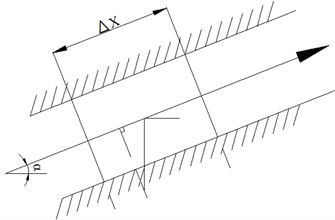Figure 1. Fluid microcluster motion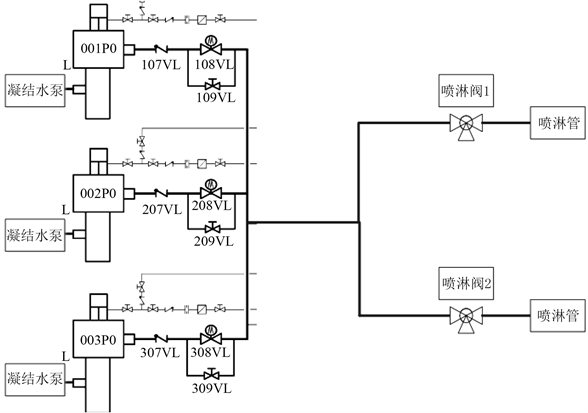Figure 2. Hydraulic modeling scope

3.2. 管道应力建模过程

4. 水力建模结果

$\Delta P\mathrm{max}=\rho c\Delta V=4.76\text{\hspace{0.17em}}\text{Mpa}$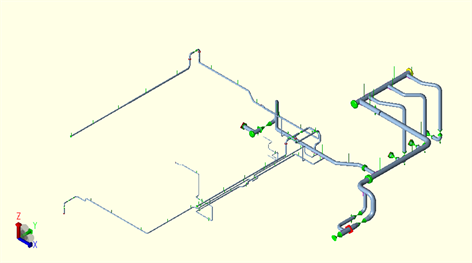Figure 3. Pipeline modeling scope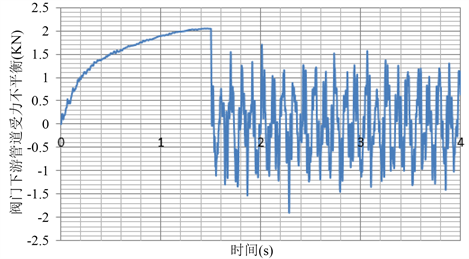Figure 4. Upstream force unbalance of spray valve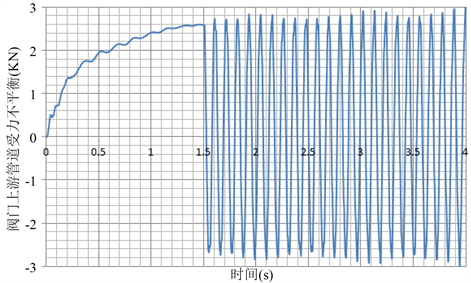Figure 5. Downstream force unbalance of spray valve

5. 管道应力建模结果

6. 改进方案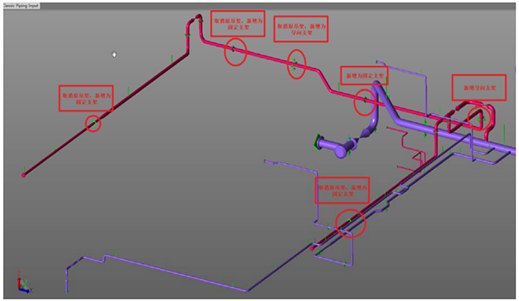Figure 6. Increased support positions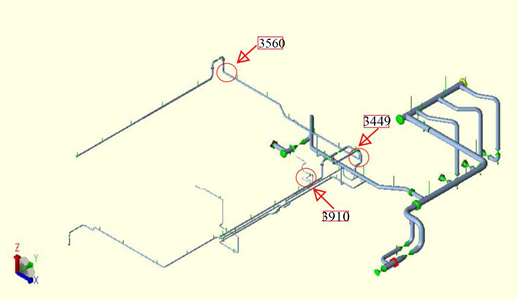Figure 7. Maximum stress pointsTable 3. Pipeline stress result after increased supportTable 4. Maximum stress points comparison before and after reforming

7. 结论

  胡晓阳. 基于Flowmaster的泵站系统水力过渡过程研究[D]: [硕士学位论文]. 成都: 西华大学, 2012.  张正楼, 张献娟, 刘玉璐. 基于Flowmaster的核电站循环冷却水系统泵出口阀关闭规律优化研究[J]. 给水排水, 2015, 51(2): 121-124.  杨勇, 沈良洁. 常规岛给水系统瞬态分析研究[J]. 产业与科技论坛, 2017, 16(18): 49-52.  王鑫, 韩伟实. 压水堆核电厂主给水管道水锤计算及分析[J]. 原子能科学技术, 2010, 44(S1): 192-197.  赵嘉明. 核动力装置一回路水锤研究及仿真计算[D]: [硕士学位论文]. 哈尔滨: 哈尔滨工程大学, 2008.  广东核电培训中心, 编. 900MW压水堆核电站系统与设备[M]. 北京: 原子能出版社, 2005.